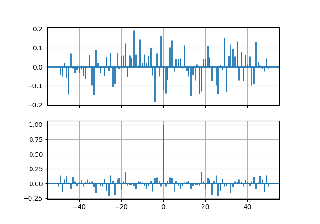# matplotlib.axes.Axes.acorr¶

Axes.acorr(self, x, *, data=None, **kwargs)[source]

Plot the autocorrelation of x.

Parameters: xarray-like detrendcallable, default: mlab.detrend_none (no detrending)A detrending function applied to x. It must have the signature detrend(x: np.ndarray) -> np.ndarray  normedbool, default: TrueIf True, input vectors are normalised to unit length. usevlinesbool, default: TrueDetermines the plot style. If True, vertical lines are plotted from 0 to the acorr value using Axes.vlines. Additionally, a horizontal line is plotted at y=0 using Axes.axhline. If False, markers are plotted at the acorr values using Axes.plot. maxlagsint, default: 10Number of lags to show. If None, will return all 2 * len(x) - 1 lags. lagsarray (length 2*maxlags+1)The lag vector. carray (length 2*maxlags+1)The auto correlation vector. lineArtist added to the axes of the correlation: LineCollection if usevlines is True. Line2D if usevlines is False. bLine2D or NoneHorizontal line at 0 if usevlines is True None usevlines is False. linestyleLine2D property, optionalThe linestyle for plotting the data points. Only used if usevlines is False. markerstr, default: 'o'The marker for plotting the data points. Only used if usevlines is False. **kwargsAdditional parameters are passed to Axes.vlines and Axes.axhline if usevlines is True; otherwise they are passed to Axes.plot.

Notes

The cross correlation is performed with numpy.correlate with mode = "full".

Note

In addition to the above described arguments, this function can take a data keyword argument. If such a data argument is given, the following arguments can also be string s, which is interpreted as data[s] (unless this raises an exception): x.

Objects passed as data must support item access (data[s]) and membership test (s in data).

## Examples using matplotlib.axes.Axes.acorr¶